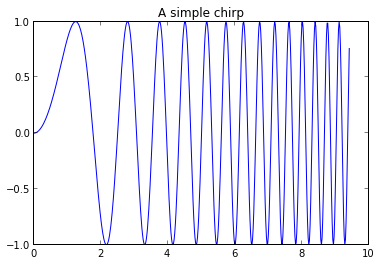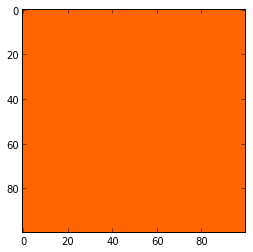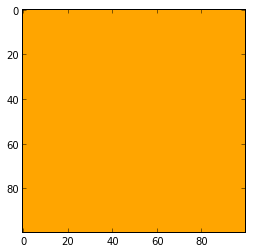IPython display hookery¶

A few niceties to help display visual output as instantly as Matplotlib plots in an IPython notebook. (To run this notebook completely, you'll need the packages reportlab and PIL to be installed.)

Plots generated with Matplotlib are shown immediately in the next cell:

In :
x = linspace(0, 3*pi, 500)
plot(x, sin(x**2))
title('A simple chirp');The same is almost true for bitmaps generated with PIL, but we have to use the convenience function imshow():

In :
from PIL import Image
from PIL.ImageDraw import Draw
img = Image.new("RGBA", (100, 100))
draw = Draw(img)
draw.rectangle(((0,0), (100, 100)), fill=(255, 100, 0))
# img.save("foo.png")
imshow(numpy.asarray(img))
Out:
<matplotlib.image.AxesImage at 0x1050da110>It would be nice to do this even more instinctively, seeing the image right after typing its name, like the interpreter shows the output of the __repr__() method, if present, as illustrated in this example:

In :
from datetime import datetime

class Foo(object):
pass

class Bar(object):

def __init__(self):
self.birthdate = datetime.now()

def __repr__(self):
bd = self.birthdate
args = (self.__class__.__name__, hex(id(self)), bd.isoformat(), bd.strftime("%A"))
return "%s instance at %s born on %s (a %s)" % args

f = Foo()
b = Bar()
In :
f
Out:
<__main__.Foo at 0x1050bda10>
In :
b
Out:
Bar instance at 0x1050bda50 born on 2013-03-14T16:38:24.088012 (a Thursday)

PIL images¶

We would like to have that immediate effect for PIL images, too! So we don't get a nasty text line like this, but the real image:

In :
from PIL import Image
from PIL.ImageDraw import Draw
img = Image.new("RGBA", (100, 100))
draw = Draw(img)
draw.rectangle(((0,0), (100, 100)), fill=(255, 100, 0))
# img.save("foo.png")
img
Out:
<PIL.Image.Image image mode=RGBA size=100x100 at 0x1050C50E0>

To get this done we just have to write an IPython display hook for the required object type (a PIL image) and register it with IPython:

In :
from io import BytesIO

from IPython.core import display
from PIL import Image

def display_pil_image(im):
"""Displayhook function for PIL Images, rendered as PNG."""

b = BytesIO()
im.save(b, format='png')
data = b.getvalue()

ip_img = display.Image(data=data, format='png', embed=True)
return ip_img._repr_png_()

# register display func with PNG formatter:
png_formatter = get_ipython().display_formatter.formatters['image/png']
dpi = png_formatter.for_type(Image.Image, display_pil_image)

Now we can just enter the identifier of a PIL image in a notebook cell, hit <ENTER> and it is shown in the next cell:

In :
from PIL import Image
from PIL.ImageDraw import Draw
img = Image.new("RGBA", (100, 100))
draw = Draw(img)
draw.rectangle(((0,0), (100, 100)), fill=(255, 100, 0))
# img.save("foo.png")
img
Out:And now for something completely different (well...)¶

Graphics can be created in many ways before it is exported into a bitmap or vector format. ReportLab Drawings are one (more) way to do that. If you don't have it installed already, now is the time to do so:

In :
!pip install reportlab
Cleaning up...

Now, again, IPython knows nothing about these drawings:

In :
from reportlab.lib import colors
from reportlab.graphics import renderPM
from reportlab.graphics.shapes import Drawing, Rect

drawing = Drawing(100, 100)
drawing.add(Rect(0, 0, 100, 100, strokeColor=None, fillColor=colors.orange))

drawing
Out:
<reportlab.graphics.shapes.Drawing instance at 0x105367c20>

Sure, we could convert a drawing to a PIL image (or even a bitmap) first, but that means one more function to remember and use (and type). And it will be likely specific to the graphics tool used:

In :
img = renderPM.drawToPIL(drawing)

img
Out:Yes, one could define a function similar to imshow() for Drawings, but that would not be very helpful if we only want to type an identifier name:

In :
drshow = lambda d: imshow(numpy.asarray(renderPM.drawToPIL(drawing)))
drshow(drawing)
Out:
<matplotlib.image.AxesImage at 0x1054f84d0>So, again, we simply want to type e.g. img and see the real thing. And, again, an IPython display hook, now for Reportlab Drawings is what we need:

In :
from io import BytesIO

from IPython.core import display

from reportlab.graphics import renderPM
from reportlab.graphics.shapes import Drawing

def display_reportlab_drawing(drawing):
"""displayhook function for ReportLab drawing, rendered as PNG"""

buff = BytesIO()
renderPM.drawToFile(drawing, buff, fmt='png', dpi=72)
data = buff.getvalue()

ip_img = display.Image(data=data, format='png', embed=True)
return ip_img._repr_png_()

# register display func with PNG formatter:
png_formatter = get_ipython().display_formatter.formatters['image/png']
drd = png_formatter.for_type(Drawing, display_reportlab_drawing)

And there we go:

In :
drawing
Out:BTW, there are some pre-built Reportlab widgets that we can see this way, too. This is illustrated here with some (only slightly modified) code taken from the testsuite:

In :
from reportlab.graphics.shapes import Drawing, Group
from reportlab.graphics.widgets.signsandsymbols import SmileyFace

def getDrawing11():

def makeSmiley(x, y, size, color):
"Make a smiley data item representation."
d = size
s = SmileyFace()
s.fillColor = color
s.x = x-d
s.y = y-d
s.size = d*2
return s

D = Drawing(400, 200)
g = Group(transform=(1,0,0,1,0,0))
g = Group(transform=(2,0,0,2,100,-100))
g = Group(transform=(2,0,0,2,0,0))
return D
In :
getDrawing11()
Out:Happy visualizing!

Exercise¶

Write a display hook for Color objects defined in reportlab.lib.colors. Try to reuse their existing __repr__() method:

In :
from reportlab.lib.colors import HexColor
c = HexColor("#ffaa00")
c
Out:
Color(1,.666667,0,1)